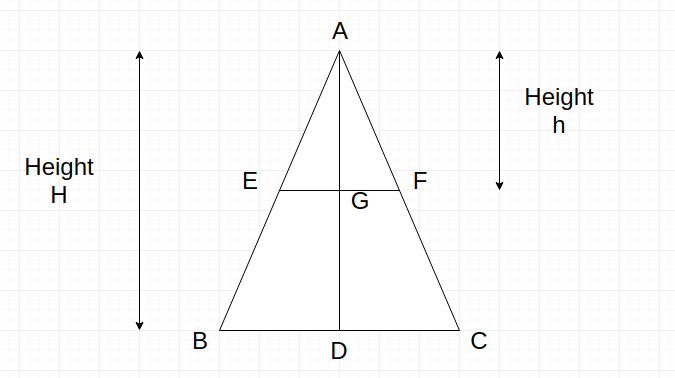# Divide an isosceles triangle in two parts with ratio of areas as n:m

Given the heightof an isosceles triangle and two integers,. The task is to find the heightfrom top of the triangle such that if we make a cut at height h from top parallel to base then the triangle must be divided into two parts with the ratio of their areas equal to n:m.

Examples:

```Input : H = 4, n = 1, m = 1
Output : 2.82843

Input : H = 4, n = 1, m = 0
Output : 4
```

## Recommended: Please try your approach on {IDE} first, before moving on to the solution.

First of all before proceeding let us mention some of the basic properties of an isosceles triangle.

Let ▲ABC is an isosceles triangle with AB = AC and BC being unequal side and base of the triangle. If D is mid-point of BC, then AD is our height H. Also, if we draw a parallel line to BC which cuts AB and AC at points E and F respectively and G being the midpoint of EF then ▲AEG is similar to ▲ABD, ▲AFG is similar to ▲ACD, ▲AEF is similar to ▲ABC, and by using properties of similar triangles we can conclude the following points:
AE/AB = AG/AD = EG/BD = EF/BC = AF/AC —–(i)As per problem’s requirement, we have to find out the height h, such that the ratio of the area of ▲AEF to the area of trapezium EFCB = n:m.

Let, h is the height of cut from the top of the triangle.
Now, area of ▲AEF = 0.5 * AG * EF and area of trapezium EFCB = 0.5 * GD * (EF+BC)
also, ratio of both is n:m.
So, we can say that ratio of area of ▲AEF to area of ▲ABC is equal to n :(n+m).
=> area of ▲AEF / area of ▲ABC = n / (n+m)
=> (0.5 * AG * EF) / (0.5 * AD * BC) = n / (n+m)
=> (AG/AD) * (EF/BC) = n / (n+m)
=> (EF/BC) * (EF/BC) = n / (n+m)
=> h2 /H2 = n / (n+m)
=> h = H*sqrt(n/(n+m))

Below is the implementation of the above approach:

## C++

 `// C++ program, to find height h ` `// which divide isosceles triangle ` `// into ratio n:m ` `#include ` `using` `namespace` `std; ` ` `  `// Function to return the height ` `float` `heightCalculate(``int` `H, ``int` `n, ``int` `m) ` `{ ` `    ``// type cast the n, m into float ` `    ``float` `N = n * 1.0; ` `    ``float` `M = m * 1.0; ` `    ``// calculate the height for cut ` `    ``float` `h = H * ``sqrt``(N / (N + M)); ` `    ``return` `h; ` `} ` ` `  `// Driver code ` `int` `main() ` `{ ` `    ``int` `H = 10, n = 3, m = 4; ` `    ``cout << heightCalculate(H, n, m); ` `    ``return` `0; ` `} `

## Java

 `// Java program, to find height h ` `// which divide isosceles triangle ` `// into ratio n:m ` ` `  `import` `java.io.*; ` ` `  `class` `GFG { ` ` `  ` `  `// Function to return the height ` `static` `float` `heightCalculate(``int` `H, ``int` `n, ``int` `m) ` `{ ` `    ``// type cast the n, m into float ` `    ``float` `N = (``float``)(n * ``1.0``); ` `    ``float` `M = (``float``)(m * ``1.0``); ` `    ``// calculate the height for cut ` `    ``float` `h = H *(``float``) Math.sqrt(N / (N + M)); ` `    ``return` `h; ` `} ` ` `  `// Driver code ` ` `  ` `  `    ``public` `static` `void` `main (String[] args) { ` `            ``int` `H = ``10``, n = ``3``, m = ``4``; ` `    ``System.out.print( heightCalculate(H, n, m)); ` `    ``} ` `} `

## Python3

 `# Python 3 program, to find height  ` `# h which divide isosceles triangle ` `# into ratio n:m ` `from` `math ``import` `sqrt ` ` `  `# Function to return the height ` `def` `heightCalculate(H, n, m): ` `     `  `    ``# type cast the n, m into float ` `    ``N ``=` `n ``*` `1.0` `    ``M ``=` `m ``*` `1.0` `     `  `    ``# calculate the height for cut ` `    ``h ``=` `H ``*` `sqrt(N ``/` `(N ``+` `M)) ` `    ``return` `h ` ` `  `# Driver code ` `if` `__name__ ``=``=` `'__main__'``: ` `    ``H ``=` `10` `    ``n ``=` `3` `    ``m ``=` `4` `    ``print``(``"{0:.6}"` `.  ` `    ``format``(heightCalculate(H, n, m))); ` `     `  `# This code is contributed  ` `# by Surendra_Gangwar `

## C#

 `// C# program, to find height h ` `// which divide isosceles triangle ` `// into ratio n:m ` `using` `System; ` ` `  `class` `GFG  ` `{ ` ` `  `// Function to return the height ` `static` `float` `heightCalculate(``int` `H, ` `                             ``int` `n, ``int` `m) ` `{ ` `    ``// type cast the n, m into float ` `    ``float` `N = (``float``)(n * 1.0); ` `    ``float` `M = (``float``)(m * 1.0); ` `     `  `    ``// calculate the height for cut ` `    ``float` `h = H * (``float``) Math.Sqrt(N / (N + M)); ` `    ``return` `h; ` `} ` ` `  `// Driver code ` `public` `static` `void` `Main () ` `{ ` `    ``int` `H = 10, n = 3, m = 4; ` `    ``Console.WriteLine(heightCalculate(H, n, m)); ` `} ` `} ` ` `  `// This code is contributed ` `// by inder_verma `

## PHP

 ` `

Output:

```6.54654
```

My Personal Notes arrow_drop_upCheck out this Author's contributed articles.

If you like GeeksforGeeks and would like to contribute, you can also write an article using contribute.geeksforgeeks.org or mail your article to contribute@geeksforgeeks.org. See your article appearing on the GeeksforGeeks main page and help other Geeks.

Please Improve this article if you find anything incorrect by clicking on the "Improve Article" button below.

Article Tags :
Practice Tags :

Be the First to upvote.

Please write to us at contribute@geeksforgeeks.org to report any issue with the above content.